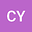Theoretical Determination of Partition of Suspended to Total Sediment Discharge
••• Chun-Yao Yang,
• Pierre Y. Julien
Chun-Yao YangThe analytical solution of Einstein's suspended load function provides us insight to the partition of suspended and total sediment discharge as well as the partition of measured and total sediment discharge. Both of the ratios can be reduced to a function of the ratio of shear velocity to the fall velocity of suspended material  $$u_*/\omega$$, flow depth $$h$$, the median grain size of bed material $$d_{50}$$. When the suspended material is fine (such as silt or clay), the ratio of suspended to total load is further reduced to a function of the ratio of flow depth to bed material size, and the ratio of measured to total load is reduced to a function of flow depth. Under this condition, at least 80% of sediment load is in suspended when  $$h/d_s > 10$$, and 90% of sediment load can be measured when $$h>1$$ m. The measurements of 35 watersheds in South Korea are used to demonstrate the proposed analysis. Furthermore, we found that for the sand bed rivers in South Korea, sediment is predominantly transported in suspension. The ratio of bedload increases when the grain size increases. When the discharge is larger than mean discharge, at least 90% of sediment is transported in suspension for gravel and sand bed rivers.